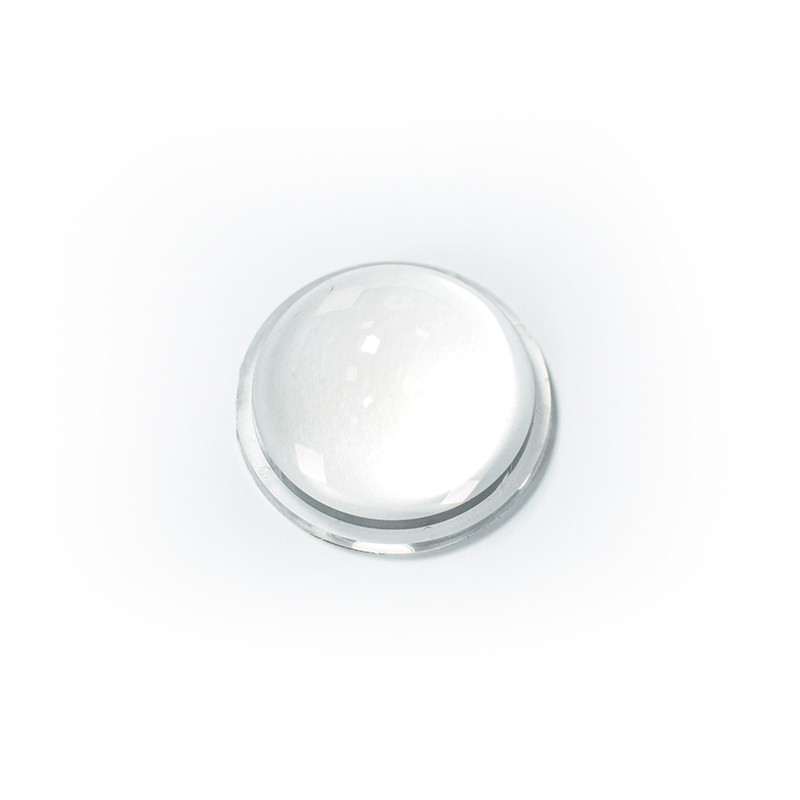# What is an LED optical lens?

Our lens is an optical element made of transparent adhesive (such as PMMA, PC, etc.). A lens is a refractor whose refracting surface is a transparent body with two spherical surfaces or one spherical surface and one plane. Its image has both real and virtual images.LED optical lenses can generally be divided into two categories: convex lenses and concave lenses. The center part is thicker than the edge part is called convex lens, there are three kinds of biconvex, plano-convex, and concave-convex; the center part is thinner than the edge part is called concave lens, and there are three kinds of bi-concave, plano-concave, and convex-concave.

Convex lens has the function of condensing light, so it is also called "converging lens" and "positive lens" (which can be used for hyperopia and reading glasses). This type of lens can be divided into: biconvex lens-a lens with both sides ground into a convex spherical surface; plano-convex lens-a lens with one convex side and a flat surface; meniscus lens-a lens with one convex and one concave.

Concave lens has the function of diverging light, so it is also called "divergent lens" and "negative lens" (which can be used for myopia glasses). This type of lens can be further divided into: double-concave lens-a lens with two sides; plano-concave lens-a lens with a concave side and a flat surface; a convex-concave lens-a lens with a convex side and a concave side.